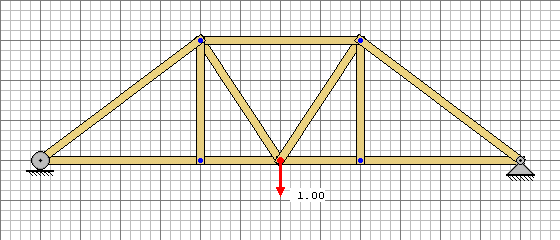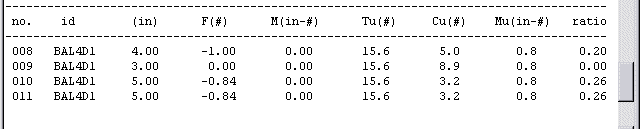# Using the Results of a Test Load Analysis

(A ModelSmart(2d) Resource)

### Problem Statement:

Use a unit test load (one unit - 1 pound) to find the probable failure load.### Solution:

Step #1 - Apply a load of 1 pound to the structure.Step #2 - Use ModelSmart to analyze the structure.

Step #3 - Locate the member(s) with the highest efficiency ratio. In this case ".26".

Step #4 - Divide the max. efficiency ratio by the actual efficiency ratio.

1/.26 = 3.8

Step #5 - Multiply by the test load.

Probable Failure Load = 3.8 x 1 =3.8 pounds.

### Looking behind the Magicians Back - The Math:

The math is based on a linear relationship known as "Similar Triangles" (see below).ModelSmart finds the probable failure load automatically when you select "Loads|Calculate Maximum" from the menu before analyzing.

Note:

In order for this process to work the structure must be capable of supporting the test load.